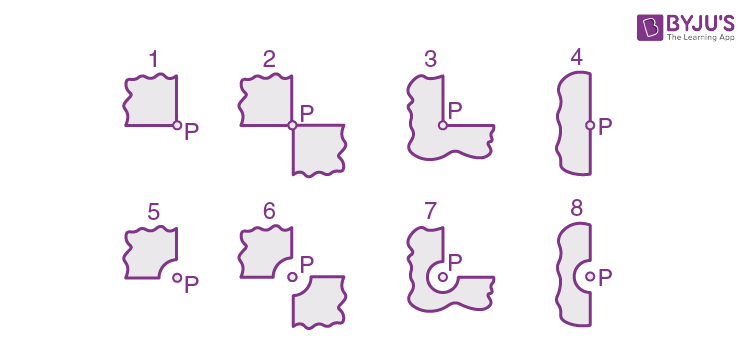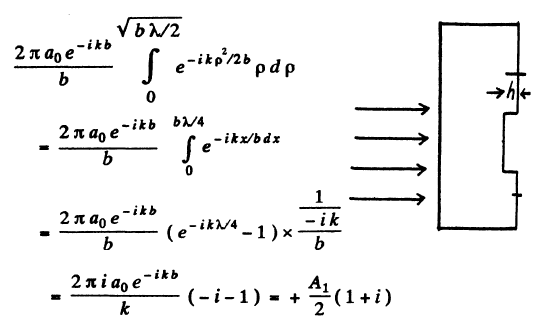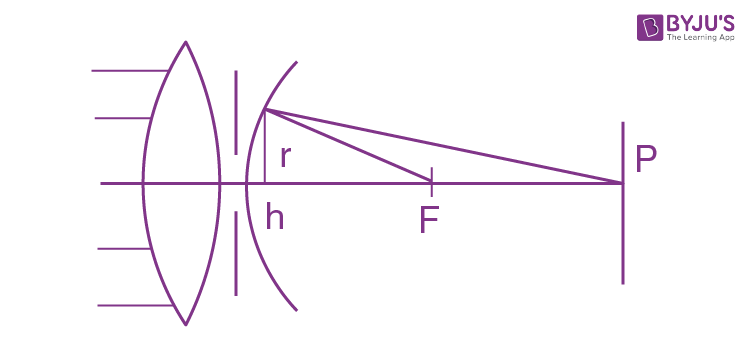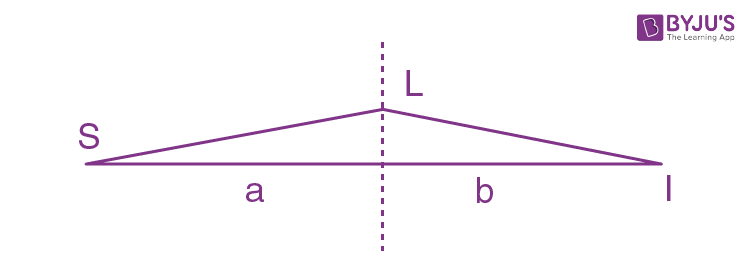Checkout JEE MAINS 2022 Question Paper Analysis : Checkout JEE MAINS 2022 Question Paper Analysis :

# I.E. Irodov Solutions on Diffraction of Light

Students can find the solutions of the Problems In General Physics I.E. Irodov - Diffraction of Light on this page. The slight bending of light when it passes around the edge of an object is called diffraction. The main topics in this chapter are the radius of the periphery of the kth Fresnel zone, Fraunhofer diffraction, angular dispersion of a diffraction grating, resolving power of a diffraction grating, resolving power of an objective, Bragg's equation, etc.

Revising these solutions will help students to improve their accuracy and problem-solving skills. Students are recommended to go through these solutions thoroughly before the exams to score higher ranks in any entrance exams.

### I.E. Irodov Solutions on Diffraction of Light

1. A plane light wave falls normally on a diaphragm with round aperture opening the first N Fresnel zones for a point P on a screen located at a distance b from the diaphragm. The wavelength of light is equal to λ. Find the intensity of light I0 in front of the diaphragm if the distribution of intensity of light I (r) on the screen is known. Here r is the distance from the point P.

Solution:

1. The radius of the periphery of the Nth Fresnel zone is

rN = √(Nbλ)

Then by conservation of energy

I0π(√(Nbλ)2 =

$$\int_{0}^{\infty }2\pi r\: drI(r)$$

Here r is the distance from the point P.

Thus I0 =

$$\frac{2}{Nb\lambda }\int_{0}^{\infty } r\: drI(r)$$

2. A point source of light with wavelength λ = 0.50 μm is located at a distance a = 100 cm in front of a diaphragm with round aperture of radius r = 1.0 mm. Find the distance b between the diaphragm and the observation point for which the number of Fresnel zones in the aperture equals k = 3.

Solution:

1. By definition rk2 = kabλ/(a + b)

for the periphery of the kth zone.

Then ark2 + brk2 = kabλ

So b = ark2/(kaλ - rk2)

= ar2/(kaλ - r2)

= 2 m

On putting the values (it is given that r = rk) for k = 3).

3. A diaphragm with round aperture, whose radius r can be varied during the experiment, is placed between a point source of light and a screen. The distances from the diaphragm to the source and the screen are equal to a = 100 cm and b = 125 cm. Determine the wavelength of light if the intensity maximum at the centre of the diffraction pattern of the screen is observed at r1 = 1.00 mm and the next maximum at r2 = 1.29 mm.

Solution:

1. Suppose maximum intensity is obtained when the aperture contains k zones. Then a minimum will be obtained for k + 1 zones. Another maximum will be obtained for k + 2 zones. Hence

r12 = kλab/(a + b)

r22 = (k+2)λab/(a + b)

Thus λ = (a + b)(r22 - r12)/2ab

= 0.598 μm (on putting the values)

4. A plane monochromatic light wave with intensity I0 falls normally on an opaque screen with a round aperture. What is the intensity of light I behind the screen at the point for which the aperture

(a) is equal to the first Fresnel zone; to the internal half of the first zone;

(b) was made equal to the first Fresnel zone and then half of it was closed (along the diameter)?

Solution:

1. (a) When the aperture is equal to the first Fresnel Zone.

The amplitude is A1 and should be compared with the amplitude A/2 when the aperture is very wide. If I0 is the intensity in the second case the intensity in the first case will be 4I0.

When the aperture is equal to the internal half of the first zone: Suppose Ain and Aout are the amplitudes due to the two halves of the first Fresnel zone. Clearly Ain and Aout differ in phase by π/2 because only half the Fresnel zone is involved. Also in magnitude

|Ain| = |Aout|

Then

A12 = 2|Ain|2

So |Ain|2 = A12/2

Hence following the argument of the first case. Iin = 2I0

(b) The aperture was made equal to the first Fresnel zone and then half of it was closed along a diameter. In this case the amplitude of vibration is A1/2.

Thus I = l0 .

5. A plane monochromatic light wave with intensity Io falls normally on an opaque disc closing the first Fresnel zone for the observation point P. What did the intensity of light I at the point P become equal to after

(a) half of the disc (along the diameter) was removed;

(b) half of the external half of the first Fresnel zone was removed (along the diameter)?

Solution:

1. (a) Suppose the disc does not obstruct light at all. Then

(because the disc covers the first Fresnel zone only).

Hence the amplitude when half of the disc is removed along a diameter

= 0

Hence I = 0

(b) In this case A = ½ Aexternal + Aremainder

= ½ ½ Aexternal - Adisc

We write Adisc = Ain + iAout

where Ain (Aout) stands for Ainternal(Aexternal). The factor i takes account of the π/2 phase difference between two halves of the first Fresnel zone. Thus

A = -½ Ain and I = ¼ Ain2

On the other hand I0 = ¼ (Ain2 + Aout2)

= ½ Ain2

So I = ½ I0.

6. A plane monochromatic light wave with intensity I0 falls normally on the surfaces of the opaque screens shown in Fig. 5.20. Find the intensity of light I at a point P.

(a) located behind the corner points of screens 1-3 and behind the edge of half-plane 4;

(b) for which the rounded-off edge of screens 5-8 coincides with the boundary of the first Fresnel zone. Derive the general formula describing the results obtained for screens 1-4; the same, for screens 5-8.Solution:

1. When the screen is fully transparent, the amplitude of vibrations in ½ A1

(with intensity I0 = ¼ A12)

(a) (1) In this case A = ¾ (½ A1) so squaring I = 9I0/16.

(2) In this case ½ of the plane is blacked out so

A = ½ (½ A1) and I = ¼ I0

(3) In this case A = ¼ (A1/2)and I = (1/16)I0

(4) In this case A = ½ (A1/2) again and I = I0/4 so I4 = I/2

In general we get I(φ) = I0(1 - (φ/2π))2

Where φ is the total angle blocked out by the screen.

(b) (5) Here A = ¾ (½ A1) + ¼ A1

A1 being the contribution of the first fresnel zone.

Thus A = ⅝ A1 and I = (25/16)I0

(6) A = ½ (½ A1) + ½ A1 = ¾ A1 and I = (9/4) I0

(7) A = ¼ (½ A1) + ¾ A1 = ⅞ A1 and I = (49/16) I0

(8) A = ½ (½ A1) + ½ A1 = ¾ A1 and I = (9/4) I0 (I8 = I6)

In 5 to 8 the first term in the expression for the amplitude is the contribution of the plane part and the second term gives the expression for the Fresnel zone part. In general in (5) to (8)

I = I0(1 + (φ/2π))2 when φ is the angle covered by the screen.

7. A plane light wave with wavelength λ and intensity I0 falls normally on a large glass plate whose opposite side serves as an opaque screen with a round aperture equal to the first Fresnel zone for the observation point P. In the middle of the aperture, there is a round recess equal to half the Fresnel zone. What must the depth h of that recess be for the intensity of light at the point P to be the highest? What is this intensity equal to?

Solution:

1. The contribution to the wave amplitude of the inner half zone isWith phase factor this becomes (A1/2)(1 + i)ewhere δ = 2π/λ)(n - 1)h. The contribution of the remaining aperture is (A1/2)(1 - i) (so that the sum of the two parts when δ = 0 is A1)

Thus the complete amplitude is

(A1/2)(1 + i)e + (A1/2)(1 - i)

And the intensity is

I = I0[(1 + i)e + (1 - i)][(1 - i)e-iδ + (1 + i)]

= I0[2 + 2 + (1 - i)2e-iδ + (1 + i)2e]

= I0[4 - 2 ie-iδ + 2ie]

= I0(4 - 4 sin δ)

Here I02 = A12/4 is the intensity of the incident light which is the same as the intensity due to an aperture of infinite extent (and no recess). Now

l is maximum when sin δ = -1

Or δ = 2kπ + 3π/2

So h = λ(k + ¾)/(n - 1)

And (b) Imax = 8I0.

8. A plane light wave with wavelength λ = 0.57 μm falls normally on a surface of a glass (n = 1.60) disc which shuts one and a half Fresnel zones for the observation point P. What must the minimum thickness of that disc be for the intensity of light at the point P to be the highest? Take into account the interference of light on its passing through the disc.

Solution:

1. We find that the contribution of the first Fresnel zone is

A1 = -(4πi/k)a0e-ikb

For the next half zone it is -(A2/2)(1 + i)

(The contribution of the remaining part of the 2nd Fresnel zone will be -(A2/2)(1 - i)

If the disc has a thickness h, the extra phase difference suffered by the light wave in passing through the disc will be

δ = (2π/λ)(n - 1)h

Thus the amplitude at P will be

Ep = (A1 - (A2/2)(1 + i))e-iδ - (A2/2)(1 - i) + A3 - A4 - A5 + …

≈ (A1(1 - i)/2)e-iδ + iA1/2

= (A1/2)(1 - i)e-iδ + i]

The corresponding intensity will be I = I0(3 - 2 cos δ - 2 sin δ)

= I0(3 - 2√2 sin (δ + π/4))

The intensity will be a maximum when

sin (δ + π/4) = -1

Or (δ + π/4) = 2kπ + 3π/2

i.e. δ = (k + ⅝). 2π

h = (λ/(n - 1))(k + ⅝), k = 0, 1, 2 ..

Note: It is not clear why k = 2 for hmin. The normal choice will be k = 0. If we take k = 0 we get hmin = 0.59 μm.

9. A plane light wave with wavelength λ = 0.54 μm goes through a thin converging lens with focal length f = 50 cm and an aperture stop fixed immediately after the lens, and reaches a screen placed at a distance b = 75 cm from the aperture stop. At what aperture radii has the centre of the diffraction pattern on the screen the maximum illuminance?

Solution:

1.Here the focal point acts as a virtual source of light. This means that we can take spherical waves converging towards F. Let us divide these waves into Fresnel zones just after they emerge from the stop. We write

r2 = f2 - (f - h)2 = (b - mλ/2)2 - (b - h)2

Here r is the radius of the mth fresnel zone and h is the distance to the left of the foot of the perpendicular. Thus

r2 = 2fh

= -bmλ + 2bh

So h = bmλ/2(b - f)

And r2 = fbmλ/(b - f)

The intensity maxima are observed when an odd number of Fresnel zones are exposed by the stop. Thus rk = √(kbfλ/(b - f)) where k = 1, 3, 5,...

10. A plane monochromatic light wave falls normally on a round aperture. At a distance b = 9.0 m from it there is a screen showing a certain diffraction pattern. The aperture diameter was decreased η = 3.0 times. Find the new distance b' at which the screen should be positioned to obtain the diffraction pattern similar to the previous one but diminished η times.

Solution:

1. For the radius of the periphery of the k th zone we have

rk = √(kλab/(a + b))

= √(kλb) if a = ∞

If the aperture diameter is reduced η times it will produce a similar diffraction pattern (reduced η times) if the radii of the Fresnel zones are also η times less. Thus

r’k = rk

This requires b’ = b/η2.

11. An opaque ball of diameter D = 40 mm is placed between a source of light with wavelength λ = 0.55 μm and a photographic plate. The distance between the source and the ball is equal to a = 12 m and that between the ball and the photographic plate is equal to b = 18 m. Find:

(a) the image dimension y' on the plate if the transverse dimension of the source is y = 6.0 mm;

(b) the minimum height of irregularities, covering the surface of the ball at random, at which the ball obstructs light.

Note. As calculations and experience show, that happens when the height of irregularities is comparable with the width of the Fresnel zone along which the edge of an opaque screen passes.

Solution:

1. (a) If a point source is placed before an opaque ball, the diffraction pattern consists of a bright spot inside a dark disc followed by fringes. The bright spot is on the line joining the point source and the centre of the ball. When the object is a finite source of transverse dimension y,

every point of the source has its corresponding image on the line joining that point and the centre of the ball. Thus the transverse dimension of the image is given by

y’ = by/a = 9mm.

(b) The minimum height of the irregularities covering the surface of the ball at random, at which the ball obstructs light is according to the note at the end of the problem, comparable with the width of the Fresnel zone along which the edge of opaque screen passes.

So hmin = ∆r

To find ∆r we note that

r2 = kλab/(a + b)

Where D = diameter of the disc (= diameter of the last Fresnel zone) and ∆k = 1.

Thus hmin = λab/D(a + b)

= 0.099 mm.

12. A point source of monochromatic 0 0 o light is positioned in front of a zone plate 0 000

1/1 at a distance a = 1.5 m from it. The image of the source is formed at a distance b = 1.0 m from the plate. Find the focal length of the zone plate.

Solution:

1. In a zone plate an undarkened circular disc is followed by a number of alternately undarkened and darkened rings. For the proper case, these correspond to 1st, 2nd, 3rd.. Fresnel zones.Ler r1 = radius of central undarkened circle. Then for this to be first Fresnel zone in the present case, we must have

SL + LI - SI = λ/2

Thus if r1 is the radius of the periphery of the first zone

√(a2 + r12) + √(b2 + r12) - (a + b) = λ/2

Or (r12/2)(1/a + 1/b) = λ/2

Or 1/a + 1/b = 1/r12

It is clear that the plate is acting as a lens of focal length f1 = r12

= ab/(a + b)

= 0.6 m

This is the principal focal length.

Other maxima are obtained when

SL + LI - SI = 3λ/2, 5λ/2,...

These focal lengths are also r12/3λ, r12/5λ,...

13. Light with wavelength λ falls on a diffraction grating at right angles. Find the angular dispersion of the grating as a function of diffraction angle θ.

Solution:

1. We have d sin θ = kλ

So dθ/dλ = D = k/d cos θ

= tan θ/λ

14. Light with wavelength λ = 589.0 nm falls normally on a diffraction grating with period d = 2.5 μm, comprising N = 10000 lines. Find the angular width of the diffraction maximum of second order.

Solution:

1. For the second-order principal maximum

d sin θ2 = 2λ = kλ

Or (Nπ/λ)d sin θ2 = 2Nπ

Minima adjacent to this maximum occur at

(Nπ/λ)d sin (θ2 ± ∆θ) = (2N± 1)π

or d cos θ2 ∆θ = λ/N

Finally angular width of the 2nd principal maximum is

2∆θ = 2λ/Nd cos θ2

= 2λ/Nd√(1 - (kλ/d)2)

= tan θ2/N

On putting the values we get 11.019’’ of arc.

15. Demonstrate that when light falls on a diffraction grating at right angles, the maximum resolving power of the grating cannot exceed the value l/λ, where l is the width of the grating and λ is the wavelength of light.

Solution:

1. Using R = λ/δλ

= kN

= Nd sin θ/λ

= l sin θ/λ

≤ l/λ

16. Using a diffraction grating as an example, demonstrate that the frequency difference of two maxima resolved according to Rayleigh's criterion is equal to the reciprocal of the difference of propagation times of the extreme interfering oscillations, i.e. δv = 1/δt.

Solution:

1. For the just resolved waves the frequency difference

δv = cδλ/λ

= c/λR

= c/λkN

= c/Nd sin θ

= 1/δt

Since Nd sin θ is the path difference between waves emitted by the extremities of the grating.

17. Light composed of two spectral lines with wavelengths 600.000 and 600.050 nm falls normally on a diffraction grating 10.0 mm wide. At a certain diffraction angle θ these lines are close to being resolved (according to Rayleigh's criterion). Find θ .

Solution:

1. δλ = 0.050 nm

R = λ/δλ

≅ 600/0.05 = 12000 (nearly)

= kN

On the other hand d sin θ = kλ

Thus (l/KN)sin θ = λ

Where l = 10-2 metre is the width of the grating

Hence sin θ = 12000×λ/l

= 12000×600×10-7

= 0.72

Or θ = 460.

18. How wide is the base of a trihedral prism which has the same resolving power as a diffraction grating with 10000 lines in the second order of the spectrum if |dn/dλ| = 0.10 μm-1?

Solution:

1. b |dn/dλ| = kN

= 2×10000

b×0.10 μm-1 = 2×104

b = 2×105 μm

= 0.2 m

= 20 cm

19. There is a telescope whose objective has a diameter D = 5.0 cm. Find the resolving power of the objective and the minimum separation between two points at a distance l = 3.0 km from the telescope, which it can resolve (assume λ = 0.55 μm).

Solution:

1. Resolving power of the objective = D/1.22λ

= 5×10-2/1.22×0.55×10-6

= 7.45×104

Let (∆y)min be the minimum distance between two points at a distance of 3.0 km which the telescope can resolve. Then

(∆y)min/3×103 = 1.22λ/D

= 1/7.45×104

Or (∆y)min = 3×103/7.45×104

= 0.04026 m

= 4.03 cm

20. Calculate the minimum separation between two points on the Moon which can be resolved by a reflecting telescope with mirror diameter 5 m. The wavelength of light is assumed to be equal

to λ = 0.55 μm.

Solution:

1. The limit of resolution of a reflecting telescope is determined by diffraction from the mirror and obeys a formula similar to that from a refracting telescope. The limit of resolution is

1/R = 1.22λ/D = (∆y)min/L

Where L = distance between the earth and the moon = 384000 km

Then putting the values λ = 0.55 μm, D = 5m

We get (∆y)min = 51.6 metre.